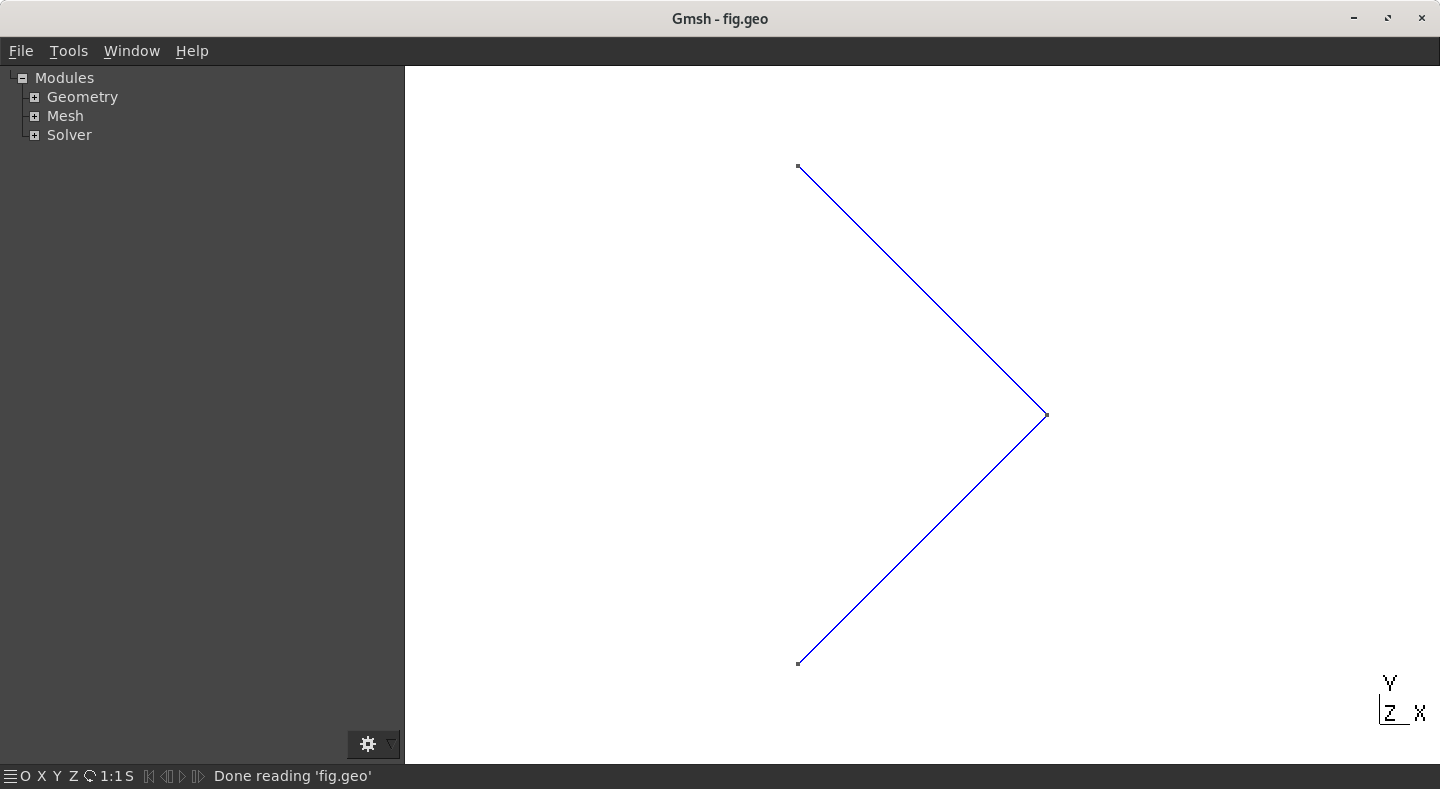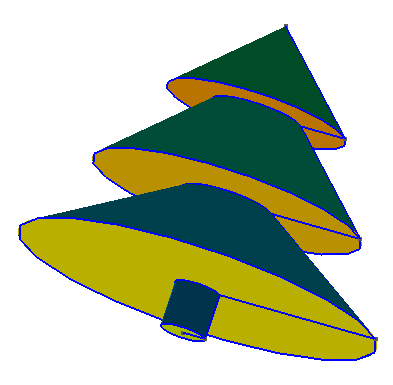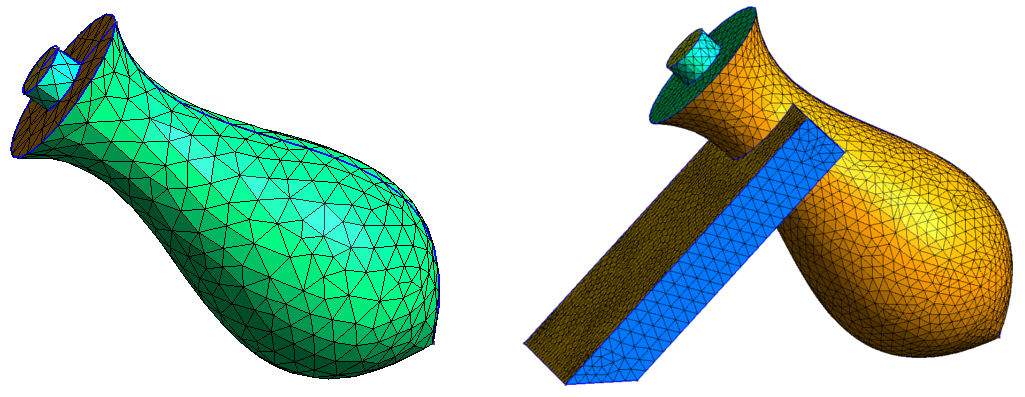# Spline and Extrusion by Rotation

## Extrusion by rotation

``````Extrude { { xr, yr, zr }, {xp, yp, zp}, alpha } { List }
``````

with (`xr`, `yr`, `zr`) a vector of the rotation axis, (`xp`, `yp`, `zp`) a point located on the axis and `alpha` the rotation angle (in rad, recal that π=`Pi`). The `List` argument contains entities to be extruded. Take a simple example:

``````SetFactory('OpenCASCADE');
h = 0.05;
Point(1) = {0,1,0,h};
Point(2) = {0.5,0.5,0,h};
Point(3) = {0,0,0,h};
Line(1) = {1,2};
Line(2) = {2,3};
``````

This code creates a broken line looking like this symbol “<":Broken line (before extrusion)

Now this brokent line is extruded by a rotation of 2π around the z-axis:

``````Extrude { {0,1,0}, {0,0,0}, 2*Pi}{Line{1,2};}
``````

This results in 2 cones, one on each other, like a diamond:Extruded broken line and meshed (2D)

By extruding a single (broken) line, draw a nice Christmas tree as on the figure below. Do not create any cone or box! The key idea is to find the line that can generate the whole geometry.Christmas tree

## Spline

A spline is a function piecewise polynomial. In GMSH, `BSpline` are `Spline` that do not necessarily cross their control points (please note that `BSpline` are not yet available with `OpenCASCADE` engine). Syntax for `Spline` and `BSpline` is the following

Syntax Definition
`Spline(indice) = {p1, p2, ..., pN}` Create a spline that goes through control `Point` `p1`,`p2`,…,`pN`
`BSpline(indice) = {p1, p2, ..., pN}` Create a B-spline thanks to control `Point` `p1`,`p2`,…,`pN`
Reminder: `BSpline` are not yet available with `OpenCASCADE`!

As usual, there is nothing more useful than an example:

``````SetFactory("OpenCASCADE");
h = 1;
Mesh.CharacteristicLengthMin = h;
Mesh.CharacteristicLengthMax = h;
Point(1) = {0,4,0,h};
Point(2) = {5,2,0,h};
Point(3) = {10,8,0,h};
Point(4) = {15,4,0,h};
Point(5) = {20,10,0,h};
Spline(1) = {1,2,3,4,5};
``````

This code create a spline:A spline

Now, extrude this spline using a 2π rotation around the x-axis

``````Extrude { {1,0,0}, {0,0,0}, 2*Pi} { Line{1} ;}
``````

And we get a cute open jar. To get a closed one, two lines should be added (on top and on bottom). The whole code would then be:

``````SetFactory("OpenCASCADE");
h = 1;
Mesh.CharacteristicLengthMin = h;
Mesh.CharacteristicLengthMax = h;
// Spline for the jar
Point(1) = {0,4,0,h};
Point(2) = {5,2,0,h};
Point(3) = {10,8,0,h};
Point(4) = {15,4,0,h};
Point(5) = {20,10,0,h};
Spline(1) = {1,2,3,4,5};
// Lines to close the jar
Point(6) = {20,0,0,h};
Point(7) = {0,0 ,0,h};
Line(2) = {5,6};
Line(3) = {7,1};
Extrude { {1,0,0}, {0,0,0}, 2*Pi} { Line{1,2,3} ;}
``````Extruded spline resuling in a jar
Using the `Visibility` window (`Tools->Visibility`) to get back indices of the surfaces, create the `Volume` associated to the jar. To do, you must add a `Surface Loop` and then the `Volume`.

## Exercise

Time to play: draw a flask using `Spline` and `Extrude`, with or without support.Flask with or without support